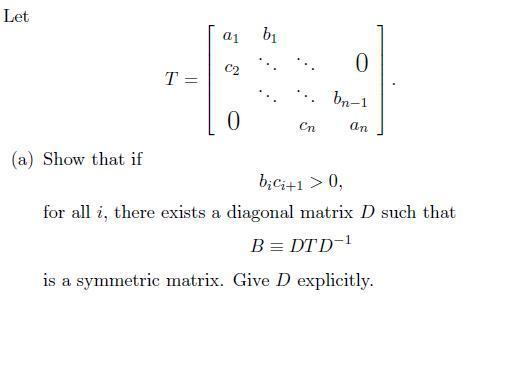# Find a diagonal matrix D such that the tridiagonal matrix T

## Homework Statement## Homework Equations

For a symmetric matrix B=B' where ' is the transpose.

## The Attempt at a Solution

Since we know that for a symmetric matrix,
B = B'

I attempted to substitude that in and tried to solve for D.

DTD-1 = (D-1)'T'D
DT = D-1T'DD
D = D-1T'DDT-1

At this point I am stumped since there are D's on both sides, what am I suppose to do?

Thanks a lot for your help.

Croco.
Code:HallsofIvy
Homework Helper
Seems to me you could just "write it out". Let x[subn[/sub] be the nth diagonal entry in D. Then 1/xn is the corresponding entry in D-1. Now, actually write out the entries for the product DTD-1. What must be true in order for that to be symmetric? If you are not sure how to do that, try it with 2 by 2 and 3 by 3 matrices first.

Notice, by the way, that saying "bici+1> 0" is exactly the same as saying "bi/ci+1> 0" or even "ci+1/bi> 0" because the only thing that is relevant is the sign: all three just say that neither of bi nor ci+1 is 0 and they have the same sign.

Hi HallsofIvy,

Thanks so much for your answer, I actually did it last night. Maybe you can have a look at my result and confirm:

In the end I got that the diagonal entries of D are:

di+1 = di$$\sqrt{b_i/c_{i+1}}$$

The square root is why bici+1 > 0

Best,
Croco